Anzeige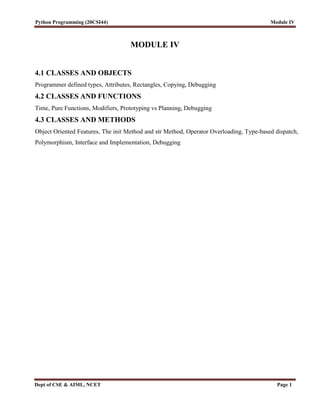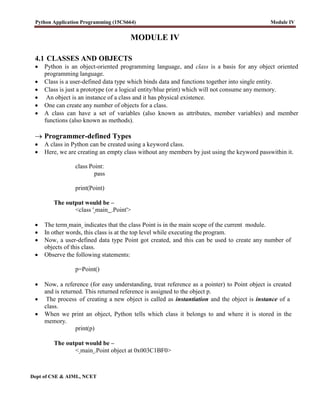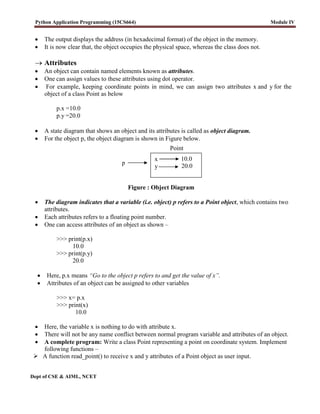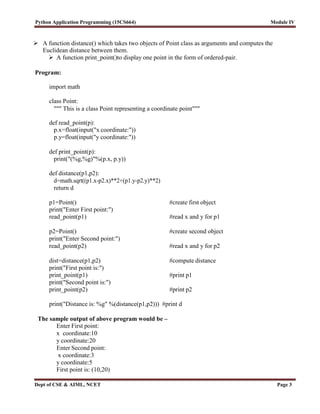Anzeige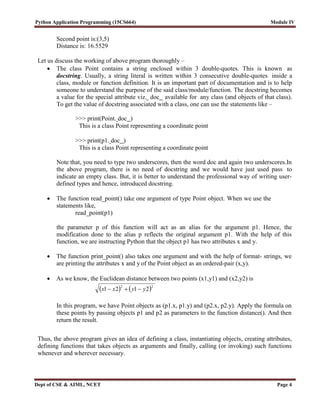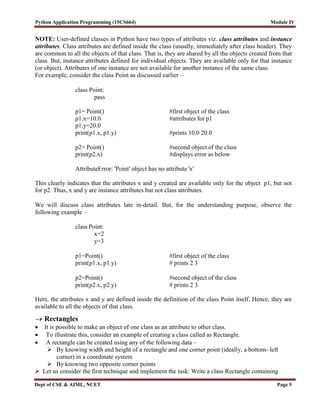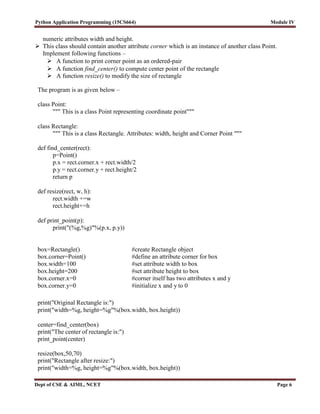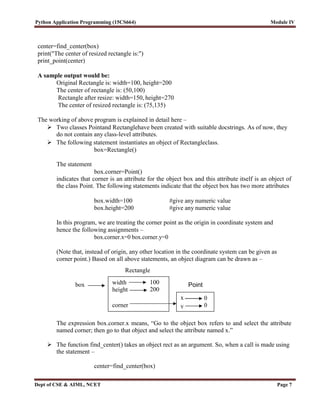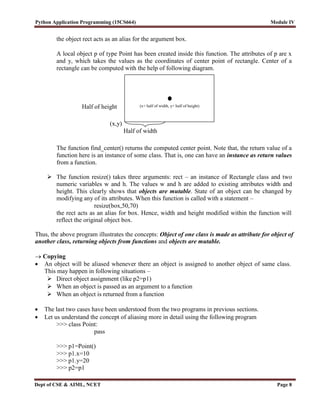Anzeige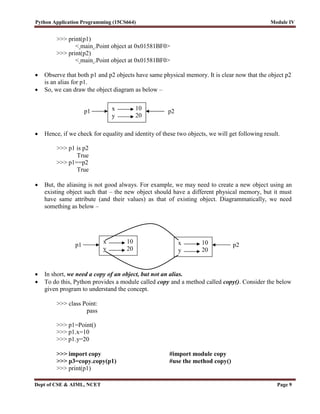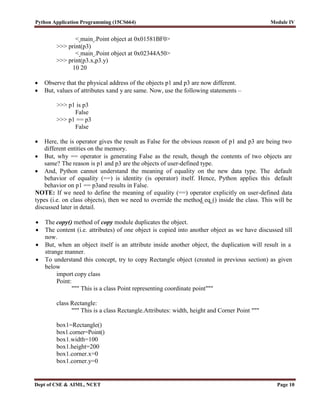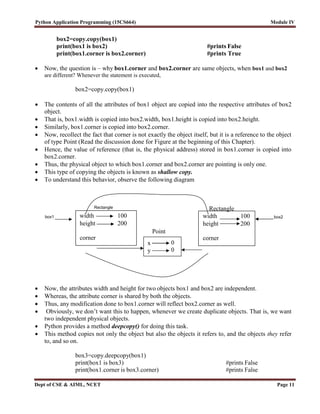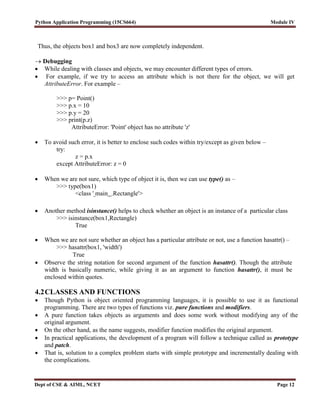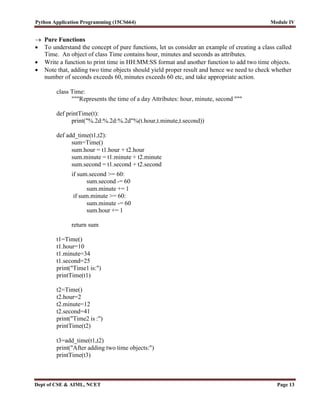Anzeige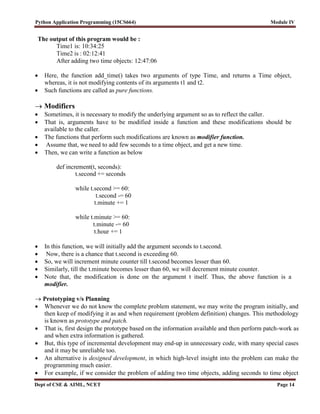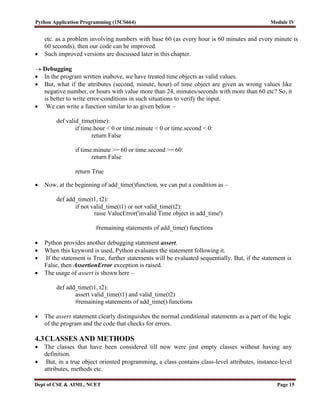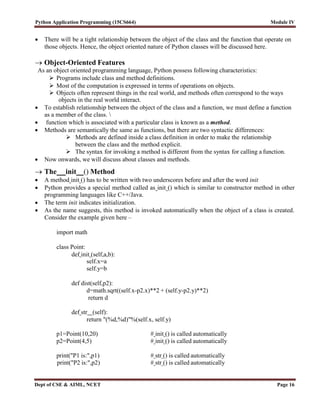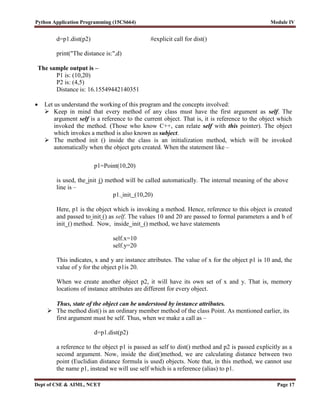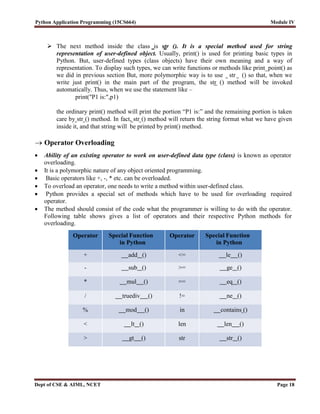Anzeige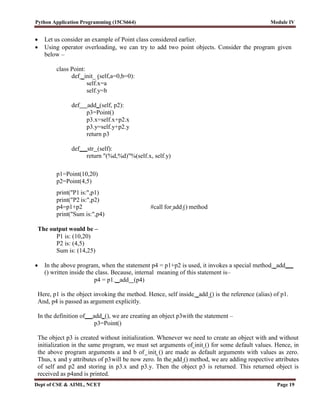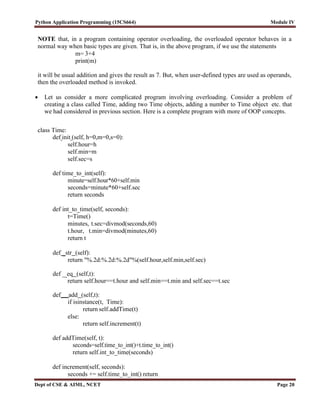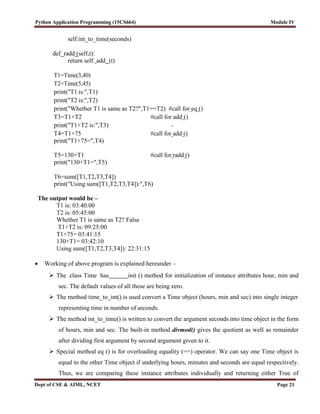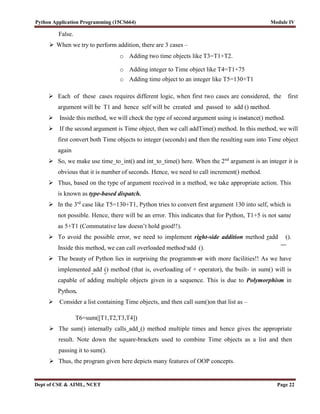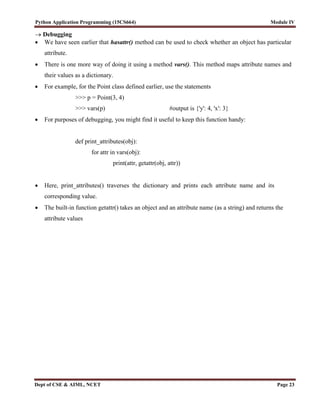Nächste SlideShare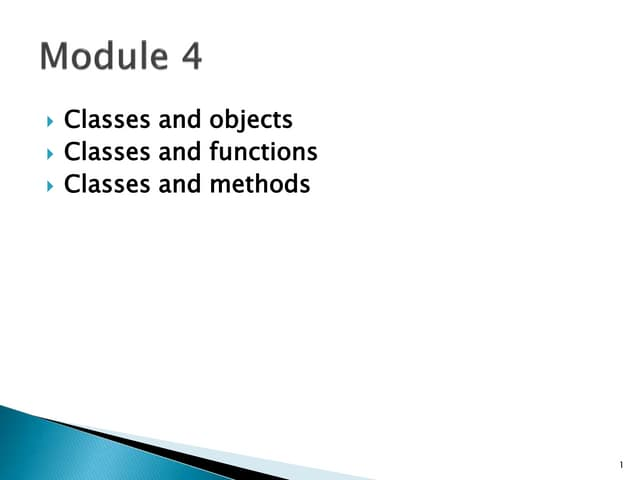Module 4.pptx
1 von 24
Anzeige

### Module IV_updated(old).pdf

1. Python Programming (20CSI44) Module IV Dept of CSE & AIML, NCET Page 1 MODULE IV 4.1 CLASSES AND OBJECTS Programmer defined types, Attributes, Rectangles, Copying, Debugging 4.2 CLASSES AND FUNCTIONS Time, Pure Functions, Modifiers, Prototyping vs Planning, Debugging 4.3 CLASSES AND METHODS Object Oriented Features, The init Method and str Method, Operator Overloading, Type-based dispatch, Polymorphism, Interface and Implementation, Debugging
2. Python Application Programming (15CS664) Module IV Dept of CSE & AIML, NCET MODULE IV 4.1 CLASSES AND OBJECTS  Python is an object-oriented programming language, and class is a basis for any object oriented programming language.  Class is a user-defined data type which binds data and functions together into single entity.  Class is just a prototype (or a logical entity/blue print) which will not consume any memory.  An object is an instance of a class and it has physical existence.  One can create any number of objects for a class.  A class can have a set of variables (also known as attributes, member variables) and member functions (also known as methods).  Programmer-defined Types  A class in Python can be created using a keyword class.  Here, we are creating an empty class without any members by just using the keyword passwithin it. class Point: pass print(Point) The output would be – <class ' main .Point'>  The term main indicates that the class Point is in the main scope of the current module.  In other words, this class is at the top level while executing the program.  Now, a user-defined data type Point got created, and this can be used to create any number of objects of this class.  Observe the following statements: p=Point()  Now, a reference (for easy understanding, treat reference as a pointer) to Point object is created and is returned. This returned reference is assigned to the object p.  The process of creating a new object is called as instantiation and the object is instance of a class.  When we print an object, Python tells which class it belongs to and where it is stored in the memory. print(p) The output would be – < main .Point object at 0x003C1BF0>
3. Python Application Programming (15CS664) Module IV Dept of CSE & AIML, NCET  The output displays the address (in hexadecimal format) of the object in the memory.  It is now clear that, the object occupies the physical space, whereas the class does not.  Attributes  An object can contain named elements known as attributes.  One can assign values to these attributes using dot operator.  For example, keeping coordinate points in mind, we can assign two attributes x and y for the object of a class Point as below p.x =10.0 p.y =20.0  A state diagram that shows an object and its attributes is called as object diagram.  For the object p, the object diagram is shown in Figure below. Point p Figure : Object Diagram  The diagram indicates that a variable (i.e. object) p refers to a Point object, which contains two attributes.  Each attributes refers to a floating point number.  One can access attributes of an object as shown – >>> print(p.x) 10.0 >>> print(p.y) 20.0  Here, p.x means “Go to the object p refers to and get the value of x”.  Attributes of an object can be assigned to other variables >>> x= p.x >>> print(x) 10.0  Here, the variable x is nothing to do with attribute x.  There will not be any name conflict between normal program variable and attributes of an object.  A complete program: Write a class Point representing a point on coordinate system. Implement following functions –  A function read_point() to receive x and y attributes of a Point object as user input. x y 10.0 20.0
4. Python Application Programming (15CS664) Module IV Dept of CSE & AIML, NCET Page 3  A function distance() which takes two objects of Point class as arguments and computes the Euclidean distance between them.  A function print_point()to display one point in the form of ordered-pair. Program: import math class Point: """ This is a class Point representing a coordinate point""" def read_point(p): p.x=float(input("x coordinate:")) p.y=float(input("y coordinate:")) def print_point(p): print("(%g,%g)"%(p.x, p.y)) def distance(p1,p2): d=math.sqrt((p1.x-p2.x)**2+(p1.y-p2.y)**2) return d p1=Point() #create first object print("Enter First point:") read_point(p1) #read x and y for p1 p2=Point() #create second object print("Enter Second point:") read_point(p2) #read x and y for p2 dist=distance(p1,p2) #compute distance print("First point is:") print_point(p1) #print p1 print("Second point is:") print_point(p2) #print p2 print("Distance is: %g" %(distance(p1,p2))) #print d The sample output of above program would be – Enter First point: x coordinate:10 y coordinate:20 Enter Second point: x coordinate:3 y coordinate:5 First point is: (10,20)
5. Python Application Programming (15CS664) Module IV Dept of CSE & AIML, NCET Page 4 x1 x22  y1 y22 Second point is:(3,5) Distance is: 16.5529 Let us discuss the working of above program thoroughly –  The class Point contains a string enclosed within 3 double-quotes. This is known as docstring. Usually, a string literal is written within 3 consecutive double-quotes inside a class, module or function definition. It is an important part of documentation and is to help someone to understand the purpose of the said class/module/function. The docstring becomes a value for the special attribute viz. doc available for any class (and objects of that class). To get the value of docstring associated with a class, one can use the statements like – >>> print(Point. doc ) This is a class Point representing a coordinate point >>> print(p1. doc ) This is a class Point representing a coordinate point Note that, you need to type two underscores, then the word doc and again two underscores.In the above program, there is no need of docstring and we would have just used pass to indicate an empty class. But, it is better to understand the professional way of writing user- defined types and hence, introduced docstring.  The function read_point() take one argument of type Point object. When we use the statements like, read_point(p1) the parameter p of this function will act as an alias for the argument p1. Hence, the modification done to the alias p reflects the original argument p1. With the help of this function, we are instructing Python that the object p1 has two attributes x and y.  The function print_point() also takes one argument and with the help of format- strings, we are printing the attributes x and y of the Point object as an ordered-pair (x,y).  As we know, the Euclidean distance between two points (x1,y1) and (x2,y2) is In this program, we have Point objects as (p1.x, p1.y) and (p2.x, p2.y). Apply the formula on these points by passing objects p1 and p2 as parameters to the function distance(). And then return the result. Thus, the above program gives an idea of defining a class, instantiating objects, creating attributes, defining functions that takes objects as arguments and finally, calling (or invoking) such functions whenever and wherever necessary.
6. Python Application Programming (15CS664) Module IV Dept of CSE & AIML, NCET Page 5 NOTE: User-defined classes in Python have two types of attributes viz. class attributes and instance attributes. Class attributes are defined inside the class (usually, immediately after class header). They are common to all the objects of that class. That is, they are shared by all the objects created from that class. But, instance attributes defined for individual objects. They are available only for that instance (or object). Attributes of one instance are not available for another instance of the same class. For example, consider the class Point as discussed earlier – class Point: pass p1= Point() #first object of the class p1.x=10.0 #attributes for p1 p1.y=20.0 print(p1.x, p1.y) #prints 10.0 20.0 p2= Point() #second object of the class print(p2.x) #displays error as below AttributeError: 'Point' object has no attribute 'x' This clearly indicates that the attributes x and y created are available only for the object p1, but not for p2. Thus, x and y are instance attributes but not class attributes. We will discuss class attributes late in-detail. But, for the understanding purpose, observe the following example – class Point: x=2 y=3 p1=Point() #first object of the class print(p1.x, p1.y) # prints 2 3 p2=Point() #second object of the class print(p2.x, p2.y) # prints 2 3 Here, the attributes x and y are defined inside the definition of the class Point itself. Hence, they are available to all the objects of that class.  Rectangles  It is possible to make an object of one class as an attribute to other class.  To illustrate this, consider an example of creating a class called as Rectangle.  A rectangle can be created using any of the following data –  By knowing width and height of a rectangle and one corner point (ideally, a bottom- left corner) in a coordinate system  By knowing two opposite corner points  Let us consider the first technique and implement the task: Write a class Rectangle containing
7. Python Application Programming (15CS664) Module IV Dept of CSE & AIML, NCET Page 6 numeric attributes width and height.  This class should contain another attribute corner which is an instance of another class Point. Implement following functions –  A function to print corner point as an ordered-pair  A function find_center() to compute center point of the rectangle  A function resize() to modify the size of rectangle The program is as given below – class Point: """ This is a class Point representing coordinate point""" class Rectangle: """ This is a class Rectangle. Attributes: width, height and Corner Point """ def find_center(rect): p=Point() p.x = rect.corner.x + rect.width/2 p.y = rect.corner.y + rect.height/2 return p def resize(rect, w, h): rect.width +=w rect.height+=h def print_point(p): print("(%g,%g)"%(p.x, p.y)) box=Rectangle() #create Rectangle object box.corner=Point() #define an attribute corner for box box.width=100 #set attribute width to box box.height=200 #set attribute height to box box.corner.x=0 #corner itself has two attributes x and y box.corner.y=0 #initialize x and y to 0 print("Original Rectangle is:") print("width=%g, height=%g"%(box.width, box.height)) center=find_center(box) print("The center of rectangle is:") print_point(center) resize(box,50,70) print("Rectangle after resize:") print("width=%g, height=%g"%(box.width, box.height))
8. Python Application Programming (15CS664) Module IV Dept of CSE & AIML, NCET Page 7 center=find_center(box) print("The center of resized rectangle is:") print_point(center) A sample output would be: Original Rectangle is: width=100, height=200 The center of rectangle is: (50,100) Rectangle after resize: width=150, height=270 The center of resized rectangle is: (75,135) The working of above program is explained in detail here –  Two classes Pointand Rectanglehave been created with suitable docstrings. As of now, they do not contain any class-level attributes.  The following statement instantiates an object of Rectangleclass. box=Rectangle() The statement box.corner=Point() indicates that corner is an attribute for the object box and this attribute itself is an object of the class Point. The following statements indicate that the object box has two more attributes box.width=100 #give any numeric value box.height=200 #give any numeric value In this program, we are treating the corner point as the origin in coordinate system and hence the following assignments – box.corner.x=0 box.corner.y=0 (Note that, instead of origin, any other location in the coordinate system can be given as corner point.) Based on all above statements, an object diagram can be drawn as – Rectangle box The expression box.corner.x means, “Go to the object box refers to and select the attribute named corner; then go to that object and select the attribute named x.”  The function find_center() takes an object rect as an argument. So, when a call is made using the statement – center=find_center(box) width height 100 200 Point x corner y 0 0
9. Python Application Programming (15CS664) Module IV Dept of CSE & AIML, NCET Page 8 (x+ half of width, y+ half of height) the object rect acts as an alias for the argument box. A local object p of type Point has been created inside this function. The attributes of p are x and y, which takes the values as the coordinates of center point of rectangle. Center of a rectangle can be computed with the help of following diagram. Half of height (x,y) Half of width The function find_center() returns the computed center point. Note that, the return value of a function here is an instance of some class. That is, one can have an instance as return values from a function.  The function resize() takes three arguments: rect – an instance of Rectangle class and two numeric variables w and h. The values w and h are added to existing attributes width and height. This clearly shows that objects are mutable. State of an object can be changed by modifying any of its attributes. When this function is called with a statement – resize(box,50,70) the rect acts as an alias for box. Hence, width and height modified within the function will reflect the original object box. Thus, the above program illustrates the concepts: Object of one class is made as attribute for object of another class, returning objects from functions and objects are mutable.  Copying  An object will be aliased whenever there an object is assigned to another object of same class. This may happen in following situations –  Direct object assignment (like p2=p1)  When an object is passed as an argument to a function  When an object is returned from a function  The last two cases have been understood from the two programs in previous sections.  Let us understand the concept of aliasing more in detail using the following program >>> class Point: pass >>> p1=Point() >>> p1.x=10 >>> p1.y=20 >>> p2=p1
10. Python Application Programming (15CS664) Module IV Dept of CSE & AIML, NCET Page 9 x y 10 20 x y 10 20 x y 10 20 >>> print(p1) < main .Point object at 0x01581BF0> >>> print(p2) < main .Point object at 0x01581BF0>  Observe that both p1 and p2 objects have same physical memory. It is clear now that the object p2 is an alias for p1.  So, we can draw the object diagram as below – p1 p2  Hence, if we check for equality and identity of these two objects, we will get following result. >>> p1 is p2 True >>> p1==p2 True  But, the aliasing is not good always. For example, we may need to create a new object using an existing object such that – the new object should have a different physical memory, but it must have same attribute (and their values) as that of existing object. Diagrammatically, we need something as below – p1 p2  In short, we need a copy of an object, but not an alias.  To do this, Python provides a module called copy and a method called copy(). Consider the below given program to understand the concept. >>> class Point: pass >>> p1=Point() >>> p1.x=10 >>> p1.y=20 >>> import copy #import module copy >>> p3=copy.copy(p1) #use the method copy() >>> print(p1)
11. Python Application Programming (15CS664) Module IV Dept of CSE & AIML, NCET Page 10 < main .Point object at 0x01581BF0> >>> print(p3) < main .Point object at 0x02344A50> >>> print(p3.x,p3.y) 10 20  Observe that the physical address of the objects p1 and p3 are now different.  But, values of attributes xand y are same. Now, use the following statements – >>> p1 is p3 False >>> p1 == p3 False  Here, the is operator gives the result as False for the obvious reason of p1 and p3 are being two different entities on the memory.  But, why == operator is generating False as the result, though the contents of two objects are same? The reason is p1 and p3 are the objects of user-defined type.  And, Python cannot understand the meaning of equality on the new data type. The default behavior of equality (==) is identity (is operator) itself. Hence, Python applies this default behavior on p1 == p3and results in False. NOTE: If we need to define the meaning of equality (==) operator explicitly on user-defined data types (i.e. on class objects), then we need to override the method eq () inside the class. This will be discussed later in detail.  The copy() method of copy module duplicates the object.  The content (i.e. attributes) of one object is copied into another object as we have discussed till now.  But, when an object itself is an attribute inside another object, the duplication will result in a strange manner.  To understand this concept, try to copy Rectangle object (created in previous section) as given below import copy class Point: """ This is a class Point representing coordinate point""" class Rectangle: """ This is a class Rectangle.Attributes: width, height and Corner Point """ box1=Rectangle() box1.corner=Point() box1.width=100 box1.height=200 box1.corner.x=0 box1.corner.y=0
12. Python Application Programming (15CS664) Module IV Dept of CSE & AIML, NCET Page 11 box2=copy.copy(box1) print(box1 is box2) #prints False print(box1.corner is box2.corner) #prints True  Now, the question is – why box1.corner and box2.corner are same objects, when box1 and box2 are different? Whenever the statement is executed, box2=copy.copy(box1)  The contents of all the attributes of box1 object are copied into the respective attributes of box2 object.  That is, box1.width is copied into box2.width, box1.height is copied into box2.height.  Similarly, box1.corner is copied into box2.corner.  Now, recollect the fact that corner is not exactly the object itself, but it is a reference to the object of type Point (Read the discussion done for Figure at the beginning of this Chapter).  Hence, the value of reference (that is, the physical address) stored in box1.corner is copied into box2.corner.  Thus, the physical object to which box1.corner and box2.corner are pointing is only one.  This type of copying the objects is known as shallow copy.  To understand this behavior, observe the following diagram Rectangle Point  Now, the attributes width and height for two objects box1 and box2 are independent.  Whereas, the attribute corner is shared by both the objects.  Thus, any modification done to box1.corner will reflect box2.corner as well.  Obviously, we don’t want this to happen, whenever we create duplicate objects. That is, we want two independent physical objects.  Python provides a method deepcopy() for doing this task.  This method copies not only the object but also the objects it refers to, and the objects they refer to, and so on. box3=copy.deepcopy(box1) print(box1 is box3) #prints False print(box1.corner is box3.corner) #prints False x y 0 0 Rectangle box1 width 100 height corner 200 width 100 box2 height corner 200
13. Python Application Programming (15CS664) Module IV Dept of CSE & AIML, NCET Page 12 Thus, the objects box1 and box3 are now completely independent.  Debugging  While dealing with classes and objects, we may encounter different types of errors.  For example, if we try to access an attribute which is not there for the object, we will get AttributeError. For example – >>> p= Point() >>> p.x = 10 >>> p.y = 20 >>> print(p.z) AttributeError: 'Point' object has no attribute 'z'  To avoid such error, it is better to enclose such codes within try/except as given below – try: z = p.x except AttributeError: z = 0  When we are not sure, which type of object it is, then we can use type() as – >>> type(box1) <class ' main .Rectangle'>  Another method isinstance() helps to check whether an object is an instance of a particular class >>> isinstance(box1,Rectangle) True  When we are not sure whether an object has a particular attribute or not, use a function hasattr() – >>> hasattr(box1, 'width') True  Observe the string notation for second argument of the function hasattr(). Though the attribute width is basically numeric, while giving it as an argument to function hasattr(), it must be enclosed within quotes. 4.2CLASSES AND FUNCTIONS  Though Python is object oriented programming languages, it is possible to use it as functional programming. There are two types of functions viz. pure functions and modifiers.  A pure function takes objects as arguments and does some work without modifying any of the original argument.  On the other hand, as the name suggests, modifier function modifies the original argument.  In practical applications, the development of a program will follow a technique called as prototype and patch.  That is, solution to a complex problem starts with simple prototype and incrementally dealing with the complications.
14. Python Application Programming (15CS664) Module IV Dept of CSE & AIML, NCET Page 13  Pure Functions  To understand the concept of pure functions, let us consider an example of creating a class called Time. An object of class Time contains hour, minutes and seconds as attributes.  Write a function to print time in HH:MM:SS format and another function to add two time objects.  Note that, adding two time objects should yield proper result and hence we need to check whether number of seconds exceeds 60, minutes exceeds 60 etc, and take appropriate action. class Time: """Represents the time of a day Attributes: hour, minute, second """ def printTime(t): print("%.2d:%.2d:%.2d"%(t.hour,t.minute,t.second)) def add_time(t1,t2): sum=Time() sum.hour = t1.hour + t2.hour sum.minute = t1.minute + t2.minute sum.second = t1.second + t2.second if sum.second >= 60: sum.second -= 60 sum.minute += 1 if sum.minute >= 60: sum.minute -= 60 sum.hour += 1 return sum t1=Time() t1.hour=10 t1.minute=34 t1.second=25 print("Time1 is:") printTime(t1) t2=Time() t2.hour=2 t2.minute=12 t2.second=41 print("Time2 is :") printTime(t2) t3=add_time(t1,t2) print("After adding two time objects:") printTime(t3)
15. Python Application Programming (15CS664) Module IV Dept of CSE & AIML, NCET Page 14 The output of this program would be : Time1 is: 10:34:25 Time2 is : 02:12:41 After adding two time objects: 12:47:06  Here, the function add_time() takes two arguments of type Time, and returns a Time object, whereas, it is not modifying contents of its arguments t1 and t2.  Such functions are called as pure functions.  Modifiers  Sometimes, it is necessary to modify the underlying argument so as to reflect the caller.  That is, arguments have to be modified inside a function and these modifications should be available to the caller.  The functions that perform such modifications are known as modifier function.  Assume that, we need to add few seconds to a time object, and get a new time.  Then, we can write a function as below def increment(t, seconds): t.second += seconds while t.second >= 60: t.second -= 60 t.minute += 1 while t.minute >= 60: t.minute -= 60 t.hour += 1  In this function, we will initially add the argument seconds to t.second.  Now, there is a chance that t.second is exceeding 60.  So, we will increment minute counter till t.second becomes lesser than 60.  Similarly, till the t.minute becomes lesser than 60, we will decrement minute counter.  Note that, the modification is done on the argument t itself. Thus, the above function is a modifier.  Prototyping v/s Planning  Whenever we do not know the complete problem statement, we may write the program initially, and then keep of modifying it as and when requirement (problem definition) changes. This methodology is known as prototype and patch.  That is, first design the prototype based on the information available and then perform patch-work as and when extra information is gathered.  But, this type of incremental development may end-up in unnecessary code, with many special cases and it may be unreliable too.  An alternative is designed development, in which high-level insight into the problem can make the programming much easier.  For example, if we consider the problem of adding two time objects, adding seconds to time object
16. Python Application Programming (15CS664) Module IV Dept of CSE & AIML, NCET Page 15 etc. as a problem involving numbers with base 60 (as every hour is 60 minutes and every minute is 60 seconds), then our code can be improved.  Such improved versions are discussed later in this chapter.  Debugging  In the program written inabove, we have treated time objects as valid values.  But, what if the attributes (second, minute, hour) of time object are given as wrong values like negative number, or hours with value more than 24, minutes/seconds with more than 60 etc? So, it is better to write error-conditions in such situations to verify the input.  We can write a function similar to as given below – def valid_time(time): if time.hour < 0 or time.minute < 0 or time.second < 0: return False if time.minute >= 60 or time.second >= 60: return False return True  Now, at the beginning of add_time()function, we can put a condition as – def add_time(t1, t2): if not valid_time(t1) or not valid_time(t2): raise ValueError('invalid Time object in add_time') #remaining statements of add_time() functions  Python provides another debugging statement assert.  When this keyword is used, Python evaluates the statement following it.  If the statement is True, further statements will be evaluated sequentially. But, if the statement is False, then AssertionError exception is raised.  The usage of assert is shown here – def add_time(t1, t2): assert valid_time(t1) and valid_time(t2) #remaining statements of add_time() functions  The assert statement clearly distinguishes the normal conditional statements as a part of the logic of the program and the code that checks for errors. 4.3CLASSES AND METHODS  The classes that have been considered till now were just empty classes without having any definition.  But, in a true object oriented programming, a class contains class-level attributes, instance-level attributes, methods etc.
17. Python Application Programming (15CS664) Module IV Dept of CSE & AIML, NCET Page 16  There will be a tight relationship between the object of the class and the function that operate on those objects. Hence, the object oriented nature of Python classes will be discussed here.  Object-Oriented Features As an object oriented programming language, Python possess following characteristics:  Programs include class and method definitions.  Most of the computation is expressed in terms of operations on objects.  Objects often represent things in the real world, and methods often correspond to the ways objects in the real world interact.  To establish relationship between the object of the class and a function, we must define a function as a member of the class.  function which is associated with a particular class is known as a method.  Methods are semantically the same as functions, but there are two syntactic differences:  Methods are defined inside a class definition in order to make the relationship between the class and the method explicit.  The syntax for invoking a method is different from the syntax for calling a function.  Now onwards, we will discuss about classes and methods.  The init () Method  A method init () has to be written with two underscores before and after the word init  Python provides a special method called as init () which is similar to constructor method in other programming languages like C++/Java.  The term init indicates initialization.  As the name suggests, this method is invoked automatically when the object of a class is created. Consider the example given here – import math class Point: def init (self,a,b): self.x=a self.y=b def dist(self,p2): d=math.sqrt((self.x-p2.x)**2 + (self.y-p2.y)**2) return d def str (self): return "(%d,%d)"%(self.x, self.y) p1=Point(10,20) # init () is called automatically p2=Point(4,5) # init () is called automatically print("P1 is:",p1) # str () is called automatically print("P2 is:",p2) # str () is called automatically
18. Python Application Programming (15CS664) Module IV Dept of CSE & AIML, NCET Page 17 d=p1.dist(p2) #explicit call for dist() print("The distance is:",d) The sample output is – P1 is: (10,20) P2 is: (4,5) Distance is: 16.15549442140351  Let us understand the working of this program and the concepts involved:  Keep in mind that every method of any class must have the first argument as self. The argument self is a reference to the current object. That is, it is reference to the object which invoked the method. (Those who know C++, can relate self with this pointer). The object which invokes a method is also known as subject.  The method init () inside the class is an initialization method, which will be invoked automatically when the object gets created. When the statement like – p1=Point(10,20) is used, the init () method will be called automatically. The internal meaning of the above line is – p1. init (10,20) Here, p1 is the object which is invoking a method. Hence, reference to this object is created and passed to init () as self. The values 10 and 20 are passed to formal parameters a and b of init () method. Now, inside init () method, we have statements self.x=10 self.y=20 This indicates, x and y are instance attributes. The value of x for the object p1 is 10 and, the value of y for the object p1is 20. When we create another object p2, it will have its own set of x and y. That is, memory locations of instance attributes are different for every object. Thus, state of the object can be understood by instance attributes.  The method dist() is an ordinary member method of the class Point. As mentioned earlier, its first argument must be self. Thus, when we make a call as – d=p1.dist(p2) a reference to the object p1 is passed as self to dist() method and p2 is passed explicitly as a second argument. Now, inside the dist()method, we are calculating distance between two point (Euclidian distance formula is used) objects. Note that, in this method, we cannot use the name p1, instead we will use self which is a reference (alias) to p1.